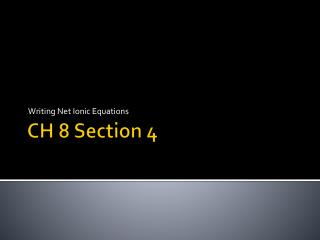DownloadDownload PresentationCH 8 Section 4

# CH 8 Section 4

Télécharger la présentation## CH 8 Section 4

- - - - - - - - - - - - - - - - - - - - - - - - - - - E N D - - - - - - - - - - - - - - - - - - - - - - - - - - -
##### Presentation Transcript

1. Writing Net Ionic Equations CH 8 Section 4

2. Ionic Equations When ionic compounds dissolve in water, the ions separate and are independent of each other. An ionic solution is indicated in a reaction by (aq.) for aqueous solution.

3. Ionic Solutions Pb(NO3)2 (aq) Is really the separate ions Pb2+ (aq) + 2 NO3- (aq)

4. Splitting Ionic Compounds into Ions Break the following into ions: KI (aq)  NaCl (aq)  Li2SO4 (aq)  CaF2 (aq) 

5. Ionic Reactions • Aqueous ions “prefer” water to any available ions. • In a double replacement reaction, new ions “preferable” to water are made available. • One of the cations, and one of the anions react to form a compound. • The other cation and anion remain unchanged and in solution.

6. Writing Net Ionic Equations • Write the total ionic equation • Split (aq) species into ions. • Leave the ppt. (or s, l, g) • Identify spectator ions • Eliminate spectator ions • Write the remaining species as a net ionic equation.

7. Net ionic equationSilver Nitrate and Potassium Bromide AgNO3(aq) + KBr(aq)  AgBr (ppt) + KNO3(aq) Split (aq) into ions, leave (ppt) as a compound. Ag+(aq) + NO3-(aq) + K+(aq) + Br-(aq)  AgBr(ppt) + K+(aq) + NO3(aq) Identify the spectator ions (species that are unchanged) NO3- (aq) + K+ (aq) are the same on both sides of the reaction. Eliminate the spectators (cross them out), write what remains as a reaction Ag+(aq) + Br-(aq)  AgBr(ppt)